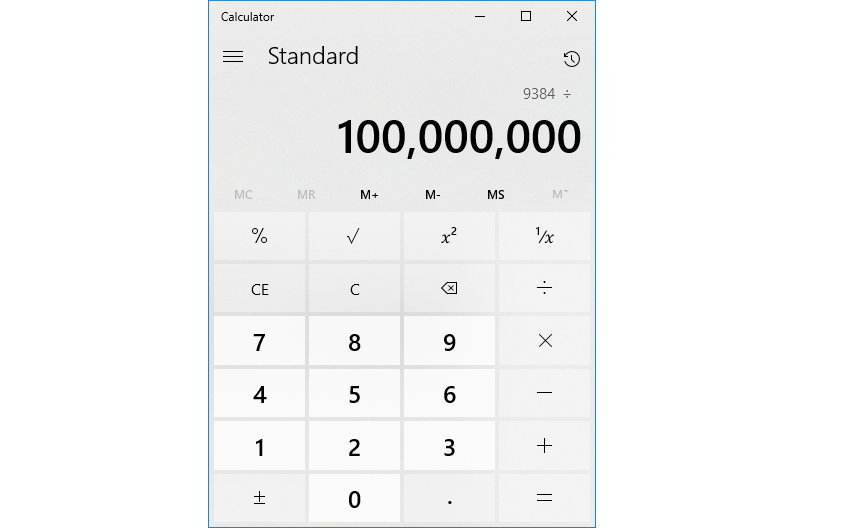比特幣單位換算指南From:
To:

另外其他常見的比特幣單位:

 一聰 = 0.00000001 ฿ 十聰 = 0.00000010 ฿ 一百聰 = 0.00000100 ฿ = 1 Bit / μBTC (you-bit) 一千聰 = 0.00001000 ฿ 一萬聰 = 0.00010000 ฿ 十萬聰 = 0.00100000 ฿ = 1 mBTC (em-bit) 一百萬聰 = 0.01000000 ฿ = 1 cBTC (bitcent) 一千萬聰 = 0.10000000 ฿ 一億聰 = 1.00000000 ฿